### Dividing Circle into Equal Parts by Concentric

Circle divided into equal parts by concentric circles.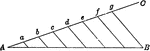### Construction of Dividing Lines

A line which is divided into seven equal parts, shown by construction.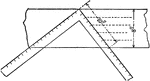### Construction of Dividing Lines

A line which is divided into equal parts, shown by construction and square.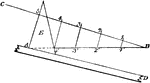### Construction Used to Divide a Line Into Equal Parts

Illustration used to show how to divide a line into any number of equal parts by construction.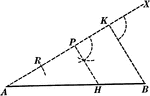### Line Divided Into Equal Parts

Illustration used to show how to divide a given straight line into any number of equal parts.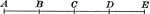### Straight Line With Points Divided Into Equal Parts

An illustration of a straight line with 5 points dividing it into 4 equal parts. Multiple of a given…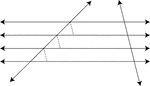### Parallel Lines Cut By A Transversal

Illustration showing that when three or more parallel lines intercept equal parts on one transversal,…